# Math Vocabulary Worksheets

#### Math Vocabulary You Should Know

There is a certain set of math vocabulary that everyone should be aware of after they have passed their secondary school. Math vocabulary comes in handy in many places and occasions. If you are giving an IQ test, that is where math vocabulary proves its importance. There is always more math vocabulary for anyone to learn; no one claim that they have learned every math vocabulary. Math vocabularies like even, and odd integers, remainders, divisors, dividends, positive and negative integers, factors, multiples, prime factors, mean, median, and mode are some common examples that everyone should be aware of. Positive integers are written on the right side of the number line, while negative integers are written on the left side of the number line. Mean, median, and mode are all average in different contexts. There are many other math vocabularies as well that are important to learn.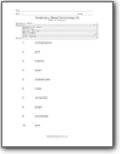###### Mixed Terminology

Riddles and puzzles on a variety of mathematics terminology. The words included in this series are: multiplication, grid, fraction, quart, ordered, pair, mass, remainder, graph, coordinate, and sum.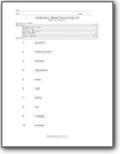###### Mixed Terminology 2

The words relate to operations and numeracy. The words included in this series are: quotient, decimal, point, division, equivalent, even, odd, factor, ten, triangle, and product.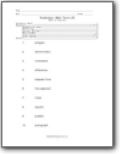###### Math Terms Pack 1

A set of nine worksheets on common mathematical terms. The words included in this series are: polygon, denominator, numerator, difference, elapsed time, line segment, mass, square, predict, and pictograph.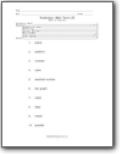###### Math Terms Pack 2

A second set of nine worksheets on common mathematical terms. The words included in this series are: plane, addition, number, cube, cardinal number, bar graph, scale, data, meter, and parallel.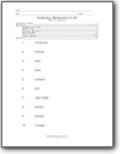###### Mathematics for All

A variety of common mathematics terms are used in nine different worksheets. The words included in this series are: horizontal, vertical, yard, even, problem, pint, right angle, sphere, division, and triangle.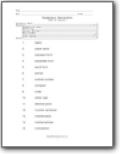###### Numeration

This set has nine worksheets integrating vocabulary terms associated with numeration. The words included in this series are: digits, place value, standard form, expanded form, word form, period, ordinal number, compare, order, dollar sign, decimal point, number sentence, mathematics, mathematician, and numeration.